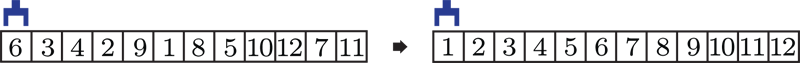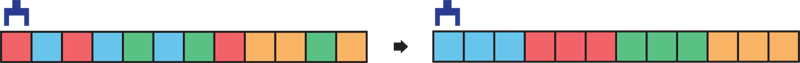# Algorithmic Robotics & Control Lab @ Rutgers

## 100 Prisoners, Cycle Following, and Rearrangement on Lattices

### The 100 Prisoners Problem

One hundred prisoners are about to be executed the next day. Their guards, feeling sorry for them or just being playful, offer them a way out but they must win a seemingly impossible challenge. Each prisoner is assigned a unique number between 1 and 100. In a windowless room, cards with numbers 1 to 100 are randomly placed in 100 closed drawers labeled from 1 to 100, one in each drawer.

The 100 prisoners will be let into the room, one by one. A prisoner in the room is allowed to open 50 drawers and look at the numbers on the cards in these drawers, with the goal to find a card with the number that matches their own. When a prisoner leaves, the room is reset to be identical before the next prisoner comes in. The prisoners may devise a strategy but may not communicate after exiting the room. They will only be set free if all prisoners can correctly find their own numbers. What are their chances?

Now, if you would like to think about the problem a bit more, you should stop reading. The answer to the 100 prinsoners problems will not be revealed here (you can find it readily via a google search), but an important clue will be.

### Cycle Following

Let us place $n$ numbers $1, \ldots, n$, in a row, from left to right, with a random ordering. We may represent this random ordering as a permtuation $\pi$, e.g., $\pi(1)$ is the number at the left most, $\pi(2)$ is the number just to the right of $\pi(1)$, and so on. Suppose these numbers are written on cards that are facing down. We can find the card with number $1$ on it by doing the following. We check the left most card, which has number $\pi(1)$. If $\pi(1) = 1$, we are done; otherwise, we check the $\pi(1)$-th card from left. What will happen as we keep doing this? We must find the card with $1$ written on it. This yields a cycle containing $1$ and possibly more numbers. For $n$ numbers that are randomly shuffled, there are about $\log n$ such cycles, which means that each cycle is of size $n/\log n$. This fact turns out to be the key for the 100 prisoners to have a decent chance of survival.

### Rearrangement on Lattices

The cycle-following procedure sketched above also applies to solving robotic rearrangement problems on lattices, as we have examined in some detail in the work

Rearrangement on Lattices with Pick-n-Swaps: Optimality Structures and
Efficient Algorithms. Jingjin Yu. R:SS 2021.


In the most basic setup, LOR (Labeled One-dimensional Rearrangement), the items are stored in a line at integer locations, out of order, and must be sorted, for example:Here, it is assumed that the robot end-effector initially rests at the leftmost end. It can pick up objects, move around, and make object swaps. The goal is to minimize the time it takes to complete the rearrangement task, assuming that each pick-n-swap operation takes a fixed amount of time and the robot end-effector travel time is proportional to end-effector travel distance (as measured by some distance metric). We assume that the pick-n-swap operation is more costly, yielding a sequential optimization problem. A solution, when executed, looks like the following:

For one dimension, partially-labeled setting is also interesting; we call this the POR (Partially-labeled One-dimensional Rearrangement) problem. In a partially-labeled problem, items of the same type/color are interchangeable. An example POR instance is as follows:An animated solution for POR looks like:

Two-dimensional versions of LOR and POR, named LTR and PTR can be posed as well. Animated solutions, which also demonstrate the problem setup, are given below.

Interested in the problem? You can find a bit more detail in the video below and if you want the full detail, you may read the paper. Let us know if you have questions!